# Texas Go Math Grade 7 Lesson 5.3 Answer Key Experimental Probability of Compound Events

Refer to our Texas Go Math Grade 7 Answer Key Pdf to score good marks in the exams. Test yourself by practicing the problems from Texas Go Math Grade 7 Lesson 5.3 Answer Key Experimental Probability of Compound Events.

## Texas Go Math Grade 7 Lesson 5.3 Answer Key Experimental Probability of Compound Events

Exploring Compound Probability

A compound event is an event that includes two or more simple events, such as flipping a coin and rolling a number cube. A compound event can include events that depend on each other or are independent. Events are independent if the occurrence of one event does not affect the probability of the other event, such as flipping a coin and rolling a number cube.

A. What are the possible outcomes of flipping a coin once?
B. What are the possible outcomes of rolling a standard number cube once?
C. Complete the list for all possible outcomes for flipping a coin and rolling a number cube.
H1, H2, ______, ______, ______, ______, T1, ______, ______, ______, ______, ______
H1 would mean the coin landed on heads, and the number cube showed a 1.
There are __________ possible outcomes for this compound event.
D. Flip a coin and roll a number cube 50 times. Use tally marks to record your results in the table.E. Based on your data, which compound event had the greatest experimental probability and what was it? The least experimental
probability?
F. Draw Conclusions Did you expect to have the same probability for each possible combination of flips and rolls? Why or why not?

Question 1.
Drink sales for an afternoon at the school carnival were recorded in the table. What is the experimental probability that the next drink is a small coffee?Total number of trials, or orders is
77 + 98 + 60 + 68 + 45 + 52 = 400
P(small coffee) =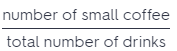Substitute 60 for number of small coffee, and 400 for total number of drinks.
P(small coffee) = $$\frac{60}{400}$$
P(small coffee) = $$\frac{3 \cdot 20}{20 \cdot 20}$$
P(small coffee) = $$\frac{3}{20}$$
The experimental probability that the next drink is a small coffee is $$\frac{3}{20}$$

Reflect

Question 2.
Make a Prediction Predict the number of cars that turn right out of 100 vehicles that enter the intersection. Explain your reasoning.
Use Proportion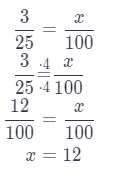You can predict that 12 out of 100 vehicles will be cars that turn right.

Question 3.
A jeweler sells necklaces made in three sizes and two different metals. Use the data from a simulation to find the experimental probability that the next necklace sold is a 20-inch gold necklace.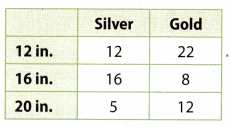P (sold necklace is a 20-inch gold necklace) =P (sold necklace is a 20-inch gold necklace) = $$\frac{4}{25}$$

Question 1.
A dentist has 400 male and female patients that range in ages from 10 years old to 50 years old and up as shown in the table. What is the experimental probability that the next patient will be female and in the age range 22-39? (Explore Activity and Example 1)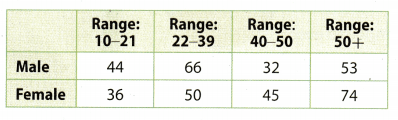Total number of trials is
44 + 66 + 32 + 53 + 36 + 50 + 45 + 74 = 400
The number of female in the age range 22 – 39 is 50The experimental probability that the next patient will be female and in the age range 22 – 39 is $$\frac{5}{40}$$.

Question 2.
At a car wash, customers can choose the type of wash and whether to use the interior vacuum. Customers are equally likely to choose each type of wash and whether to use the vacuum. Use a simulation to find the experimental probability that the next customer purchases a deluxe wash and no interior vacuum. Describe your simulation. (Example 2)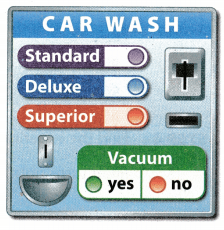Lets look at the standard cube and let each number indicate one of the possibilities:
1- Deluxe wash without interior vacuum
2-DeLuxe washing with interior vacuum
3-Standard washing with interior vacuum
4-Standard washing without interior vacuum
5-Superior washing with interior vacuum
6-Superior washing without interior vacuum
Let’s throw the cube 10 times and find the experimental probability of the number of deluxe washing with no interior vacuum
We get:
1, 5, 6, 4, 2, 1, 2, 5, 6, 3
The experimental probabiLity that the next costumers purchases a deluxe wash with no interior vacuum is $$\frac{2}{6}$$ = $$\frac{1}{3}$$.

Essential Question Check-In

Question 3.
How do you find the experimental probability of a compound event?
Experimental probability of a compound event is the number which we get when the number of times the event occurs divide by total number of trials.

Question 4.
Represent Real-World Problems For the same food trailer mentioned in Example 1, explain how to find the experimental probability that the next order is two pieces of chicken with a green salad.
Total number of trials, or orders is
33 + 22 + 52 + 35 + 13 + 55 + 65 + 55 = 330
The number of 2 piece + green salad is 33.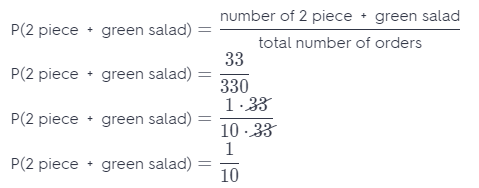The experimental probability that the next order is 2 piece + green salad is $$\frac{1}{10}$$.

The school store sells spiral notebooks in four colors and three different sizes. The table shows the sales by size and color for 400 notebooks.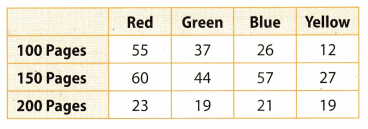Question 5.
What is the experimental probability that the next customer buys a red notebook with 150 pages?
Total number of trials, or notebooks is
55 + 37 + 26 + 12 + 60 + 44 + 57 + 27 + 23 + 19 + 21 + 19 = 400
The number of red notebook with 150 pages is 60.
number of red notebooks with 150 pagesThe experimental probability that the next customer buys a red notebook with 150 pages is $$\frac{3}{20}$$.

Question 6.
What is the experimental probability that the next customer buys any red notebook?
Total number of trials, or notebooks is
55 + 37 + 26 + 12 + 60 + 44 + 57 + 27 + 23 + 19 + 21 + 19 = 400
The number of red notebook is 138The experimental probability that the next customer buys a red notebook is $$\frac{63}{200}$$.

Question 7.
Analyze Relationships How many possible combined page count and color choices are possible? How does this number relate to the number of page size choices and to the number of color choices?
We have 3 choices for page size and 4 choices for color, therefore, we have 3 ∙ 4 = 12 possibilities to combine page size and color
Relate of number of possibilities to combine and number of choices for size is: 12 : 3 = 4 : 1
Relate of number of possibilities to combine and number of choices for color is: 12 : 4 = 3 : 1

A middle school English teacher polled random students about how many pages of a book they read per week.Question 8.
Critique Reasoning Jennie says the experimental probability that a 7th grade student reads at least 100 pages per week is $$\frac{16}{125}$$. What is her error and the correct experimental probability?
Total number of trials, or pages of book is
24 + 18 + 22 + 22 + 32 + 24 + 30 + 53 + 25 = 250
At least 100 pages means that a student reads 100 or more pages.
To the probability she calculated we have to add the probability that a 7th grade student reads 150 pages per week.
The number of 7th grade students who reads 150 pages per week is 53.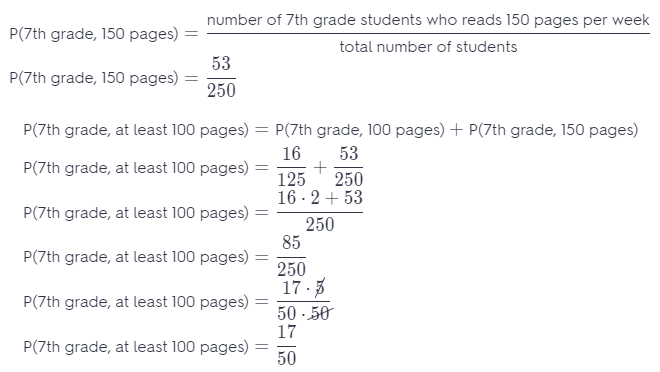The experimental probability that a 7th grade students reads at least 100 pages per week is $$\frac{17}{50}$$.

Question 9.
Analyze Relationships Based on the data, which group(s) of students should be encouraged to read more? Explain your reasoning.
Based on the data gathered for the group of students who read books, the encouragement to read more should be done for Grade 8 students. This is because they have the lowest data among the 3 groups.

H.O.T. Focus on Higher Order Thinking

Question 10.
Make a Conjecture Would you expect the probability for the simple event “rolling a 6” to be greater than or less than the probability of the compound event “rolling a 6 and getting heads on a coin”? Explain.
Total number of possible outcomes is 1, 2, 3, 4, 5, 6, therefore 6 possibilities.
P1 (rolling a 6) =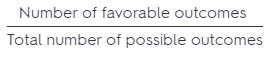= $$\frac{1}{6}$$
Total number of possible outcomes is 6 possibilities for a cube and 2 possibilities for a coin, therefore 12.
P2 (rolling a 6 and getting a head) == $$\frac{1}{12}$$
Conclusion: P1 > P2

Question 11.
Critique Reasoning Donald says he uses a standard number cube for simulations that involve 2, 3, or 6 equal outcomes. Explain how Donald can do this.
Involve two equal outcomes:
Let 6 balls in the bag, 3 white and 3 blue. If we throw the number 1, 2, or 3, we pick the bLue, otherwise we pick the white one.

Involve three equal outcomes:
Let 6 balls in the bag, 2 white, 2 blue and 2 yellow. If we throw the number 1 or 2, we pick the blue, 3 or 4 we pick the white one, otherwise wi pick a yellow ball.

Involve six equal outcomes:
Let 6 balls in the bag, white, blue yellow, red, green and orange. If we throw the number 1 we pick the blue, 2 we pick the white one, 3- yelLow, 4-green, 5-orange 6-red ball.

Question 12.
Draw Conclusions Data collected in a mall recorded the shoe styles worn by 150 male and 150 female customers. What is the probability that the next customer is male and has an open-toe shoe (such as a sandal)? What is the probability that the next male customer has an open-toe shoe? Are the two probabilities the same? Explain.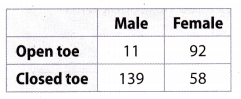Total number of trials, or customers is
11 + 92 + 139 + 58 = 300
The number of male who has an open toe shoe is 11.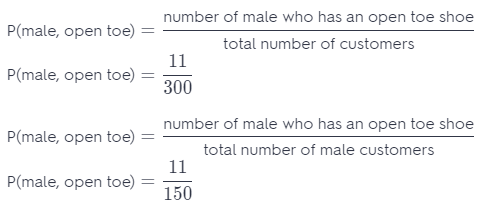No, they are not because the total number of trials are different. In the first case, we observe all customers, and in the second we only observe male customers.

Question 13.
What If? Suppose you wanted to perform a simulation to model the shoe style data shown in the table. Could you use two coins? Explain.
Yes, we can If we throw the first coin and the tails falls a male customers is watching, otherwise we observe female costumers. If we throw a second coin, and a head falls, the costumers are with open toe shoes, otherwise is closed toe shoes.

Question 14.
Represent Real-World Problems A middle school is made up of grades 6, 7, and 8, and has about the same number of male and female students in each grade. Explain how to use a simulation to find the experimental probability that the first 50 students who arrive at school are male and 7th graders.